### 8.5.1.2 Defining a feedback plan over a cover

The ideas from Section 8.4.2 can be adapted to define a feedback plan over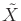using a cover. Let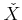denote a discrete state space in which each superstate is a neighborhood. Most of the components of the associated discrete planning problems are the same as in Section 8.4.2. The only difference is in the definition of superactions because neighborhoods can overlap in a cover. For each neighborhood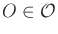, a superaction exists for each other neighborhood,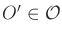such that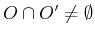(usually, their interiors overlap to yield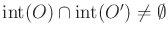).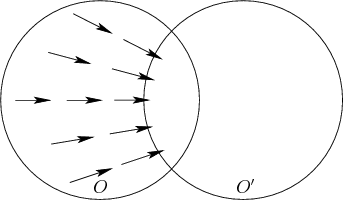Note that in the case of a cell decomposition, this produces no superactions because it is a partition. To follow the metaphor of composing funnels, the domains of some funnels should overlap, as shown in Figure 8.14. A transition from one neighborhood,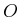, to another,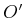, is obtained by defining a vector field onthat sends all states from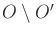into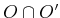; see Figure 8.16. Onceis reached, the vector field ofis no longer followed; instead, the vector field ofis used. Using the vector field of, a transition may be applied to reach another neighborhood. Note that the jump from the vector field ofto that ofmay cause the feedback plan to be a discontinuous vector field on. If the cover is designed so thatis large (if they intersect), then gradual transitions may be possible by blending the vector fields fromand.

Once the discrete problem has been defined, a discrete feedback plan can be computed over, as defined in Section 8.2. This is converted into a feedback plan overby defining a vector field on each neighborhood that causes the appropriate transitions. Each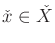can be interpreted both as a superstate and a neighborhood. For each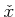, the discrete feedback plan produces a superaction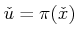, which yields a new neighborhood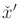. The vector field over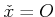is then designed to send all states into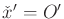.

If desired, a navigation function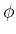overcan even be derived from a navigation function,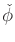, over. Suppose thatis constructed so that every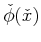is distinct for every. Any navigation function can be easily transformed to satisfy this constraint (becauseis finite). Let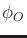denote a navigation function over some. Assume that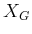is a point,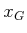(extensions can be made to more general cases). For every neighborhoodsuch that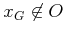,is defined so that performing gradient descent leads into the overlapping neighborhood for whichis smallest. Ifcontains, the navigation functionsimply guides the state to.

The navigation functions over eachcan be easily pieced together to yield a navigation function over all of. In places where multiple neighborhoods overlap,is defined to be the navigation function associated with the neighborhood for whichis smallest. This can be achieved by adding a large constant to each. Let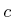denote a constant for which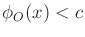over alland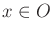(it is assumed that eachis bounded). Suppose thatassumes only integer values. Let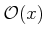denote the set of allsuch that. The navigation function overis defined as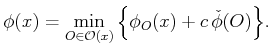(8.51)

Steven M LaValle 2020-08-14